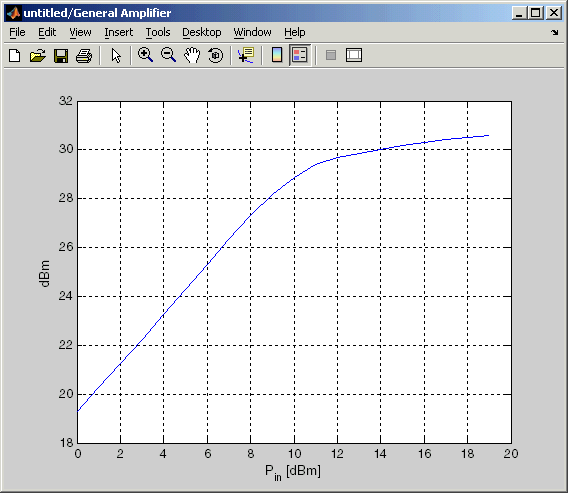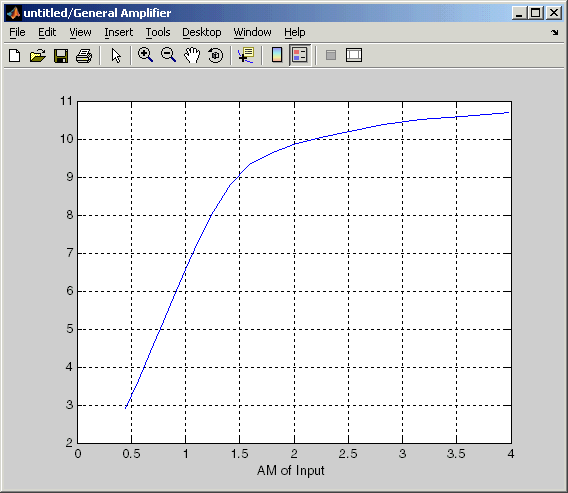# Output Port

Connection block from RF physical blocks to Simulink environment

## Library

Input/Output Ports sublibrary of the Physical library

•## Description

The Output Port block produces the baseband-equivalent time-domain response of an input signal traveling through a series of RF physical components. The Output Port block

1. Partitions the RF physical components into linear and nonlinear subsystems.

2. Extracts the complex impulse response of the linear subsystem for baseband-equivalent modeling of the RF linear system.

3. Extracts the nonlinear AMAM/AMPM modeling for RF nonlinearity.

The Output Port block also serves as a connecting port from an RF physical part of the model to the Simulink®, or mathematical, part of the model. For more information about how the Output Port block converts the physical modeling environment signals to mathematical Simulink signals, see Convert to and from Simulink Signals.

### Note

Some RF blocks require the sample time to perform baseband modeling calculations. To ensure the accuracy of these calculations, the Input Port block, as well as the mathematical RF blocks, compare the input sample time to the sample time you provide in the mask. If they do not match, or if the input sample time is missing because the blocks are not connected, an error message appears.

### Linear Subsystem

For the linear subsystem, the Output Port block uses the Input Port block parameters and the interpolated S-parameters calculated by each of the cascaded physical blocks to calculate the baseband-equivalent impulse response. Specifically, it

1. Determines the modeling frequencies f as an N-element vector. The modeling frequencies are a function of the center frequency fc, the sample time ts, and the finite impulse response filter length N, all of which you specify in the Input Port block dialog box.

The nth element of f, fn, is given by

`$\begin{array}{cc}{f}_{n}={f}_{\mathrm{min}}+\frac{n-1}{{t}_{s}N}& n=1,...,N\end{array}$`

where

`${f}_{\mathrm{min}}={f}_{c}-\frac{1}{2{t}_{s}}$`
2. Calculates the passband transfer function for the frequency range as

`$H\left(f\right)=\frac{{V}_{L}\left(f\right)}{{V}_{S}\left(f\right)}$`

where VS and VL are the source and load voltages, and f represents the modeling frequencies. More specifically,

`$H\left(f\right)=\frac{{S}_{21}\left(1+{\Gamma }_{l}\right)\left(1-{\Gamma }_{s}\right)}{2\left(1-{S}_{22}{\Gamma }_{l}\right)\left(1-{\Gamma }_{in}{\Gamma }_{s}\right)}$`

where

`$\begin{array}{l}{\Gamma }_{l}=\frac{{Z}_{l}-{Z}_{o}}{{Z}_{l}+{Z}_{o}}\\ {\Gamma }_{s}=\frac{{Z}_{s}-{Z}_{o}}{{Z}_{s}+{Z}_{o}}\\ {\Gamma }_{in}={S}_{11}+\left({S}_{12}{S}_{21}\frac{{\Gamma }_{l}}{\left(1-{S}_{22}{\Gamma }_{l}\right)}\right)\end{array}$`

and

• ZS is the source impedance.

• ZL is the load impedance.

• Sij are the S-parameters of a two-port network.

The blockset derives the passband transfer function from the Input Port block parameters as shown in the following figure:3. Translates the passband transfer function to baseband as H(f – fc), where fc is the specified center frequency.

The baseband transfer function is shown in the following figure.4. Obtains the baseband-equivalent impulse response by calculating the inverse FFT of the baseband transfer function. For faster simulation, the block calculates the IFFT using the next power of 2 greater than the specified finite impulse response filter length. Then, it truncates the impulse response to a length equal to the filter length specified.

For the linear subsystem, the Output Port block uses the calculated impulse response as input to the DSP System Toolbox™ Digital Filter Design block to determine the output.

### Nonlinear Subsystem

The nonlinear subsystem is implemented by AM/AM and AM/PM nonlinear models, as shown in the following figure.The nonlinearities of AM/AM and AM/PM conversions are extracted from the power data of an amplifier or mixer by the equations

`$\begin{array}{c}A{M}_{out}=\sqrt{{R}_{l}{P}_{out}}\\ P{M}_{out}=\phi \\ A{M}_{in}=\sqrt{{R}_{s}{P}_{in}}\end{array}$`

where AMin is the AM of the input voltage, AMout and PMout are the AM and PM of the output voltage, Rs is the source resistance (50 ohms), Rl is the load resistance (50 ohms), Pin is the input power, Pout is the output power, andϕ is the phase shift between the input and output voltage.

### Note

You can provide power data via a `.amp` file. See AMP File Data Sections (RF Toolbox) for information about this format.

The following figure shows the original power data of an amplifier.This figure shows the extracted AM/AM nonlinear conversion.## Parameters

### Main Tab

Load impedance of the RF network described in the physical model to which it connects.

### Visualization Tab

This tab shows parameters for creating plots if you display the Output Port mask after you perform one or more of the following actions:

• Run a model with two or more blocks between the Input Port block and the Output Port block.

• Click the Update Diagram button to initialize a model with two or more blocks between the Input Port block and the Output Port block.

For information about plotting, see Create Plots.

`s2y` (RF Toolbox™)